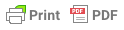The Rule of 10 and 12 is a mathematical corollary to third and fifth best leads. It enables the third hand player to count how many cards declarer holds which are higher than the opening lead.

## Rule of 10

The Rule of 10 is used when a lead is fifth-best. It works as follows:

1. Subtract the opening lead spot card from 10.

2. Also subtract the number of cards in dummy that are higher than the card led.

3. Finally, subtract the number of cards in your hand that are higher than the card led.

4. The final number equals how many higher cards declarer holds in the suit.

Here's an example:

 Partner ♥ 5 Declarer Dummy ♥ ? ♥ 10 9 6 You ♥ K J 2

Partner leads the 5. Assuming this is a fifth-best lead, how many hearts are in declarer's hand which are higher?

1. Partner's spot card is the 5, so 10 − 5 = 5.

2. Dummy contains three hearts higher than the 5, so 5 − 3 = 2.

3. You hold two hearts higher than the 5, so 2 − 2 = 0.

Thus, declarer does not have any card above the 5 if partner's lead was fifth-best. The full suit distribution around the table:

 Partner ♥ A Q 8 7 5 Declarer Dummy ♥ 4 3 ♥ 10 9 6 You ♥ K J 2

## Rule of 12

The Rule of 12 is used when a lead is third-best. It works the same as the Rule of 10, except that the starting number is 12.

Example:

 Partner ♥ 5 Declarer Dummy ♥ ?10 9 6 You ♥ K J 2

Partner leads the 5. Assuming this is a third-best lead, how many hearts are in declarer's hand which are higher?

1. Partner's spot card is the 5, so 12 − 5 = 7.

2. Dummy contains three hearts higher than the 5, so 7 − 3 = 4.

3. You hold two hearts higher than the 5, so 4 − 2 = 2.

Thus, declarer holds two hearts higher than the 5 if partner's lead was third-best. The full suit distribution around the table:

 Partner ♥ Q 8 5 4 Declarer Dummy ♥ A 7 3 ♥ 10 9 6 You ♥ K J 2